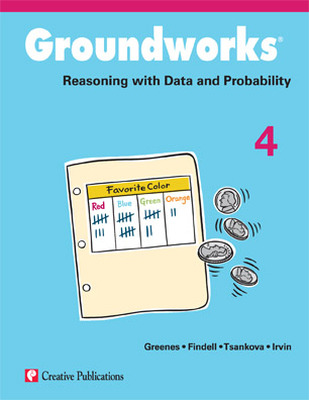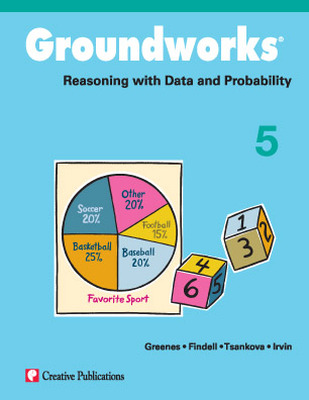# Groundworks Reasoning with Data and Probability

Groundworks: Reasoning with Data and Probability helps students develop their ability to interpret and reason with data analysis and probability. Across all ages and grades, students are continually confronted with the displays of data to interpret. The goal of this text is to help students interpret displays of data, organize data, and describe data while introducing them to the concepts of probability. Each Groundworks title is available in a separate book for grades 1-7 and contains reproducible pages.Big Ideas

Just like the other titles in the Groundworks series, Reasoning with Data and Probability centers on the big ideas of data organization and analysis and probability using interesting and challenging problems. The five big ideas in Reasoning with Data and Probability are:

• Interpret Displays of Data
• Organize Data
• Describe Data
• Ways to Count
• Probability

Text Organization

The text contains 12 different sets of problems. A set refers to a specific type of mathematical reasoning problem. Each set contains six different problems for ample practice reinforcement. With 12 different sets of six problems, each text totals 72 different mathematical reasoning problems.

Problem Sets

Each problem set consists of eight pages and begins with a teaching information page. This age contains several features to help guide the teacher through the activity, either as a class or individually. Following the teacher page are six pages of student problems, each containing one problem, all addressing the same concept. The final page in the problem set is a solutions page.

## Program Components

•Groundworks: Reasoning with Data and Probability, Grade 4
•Groundworks: Reasoning with Data and Probability, Grade 5
Sort by: